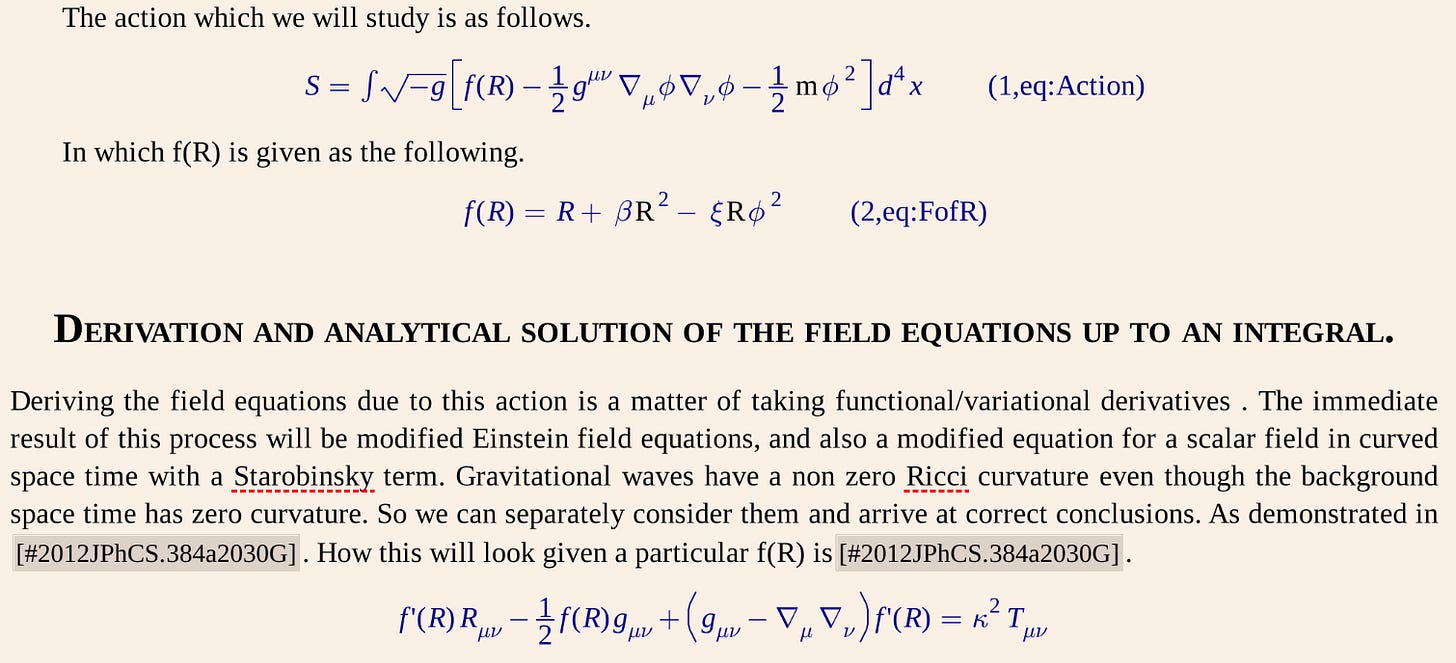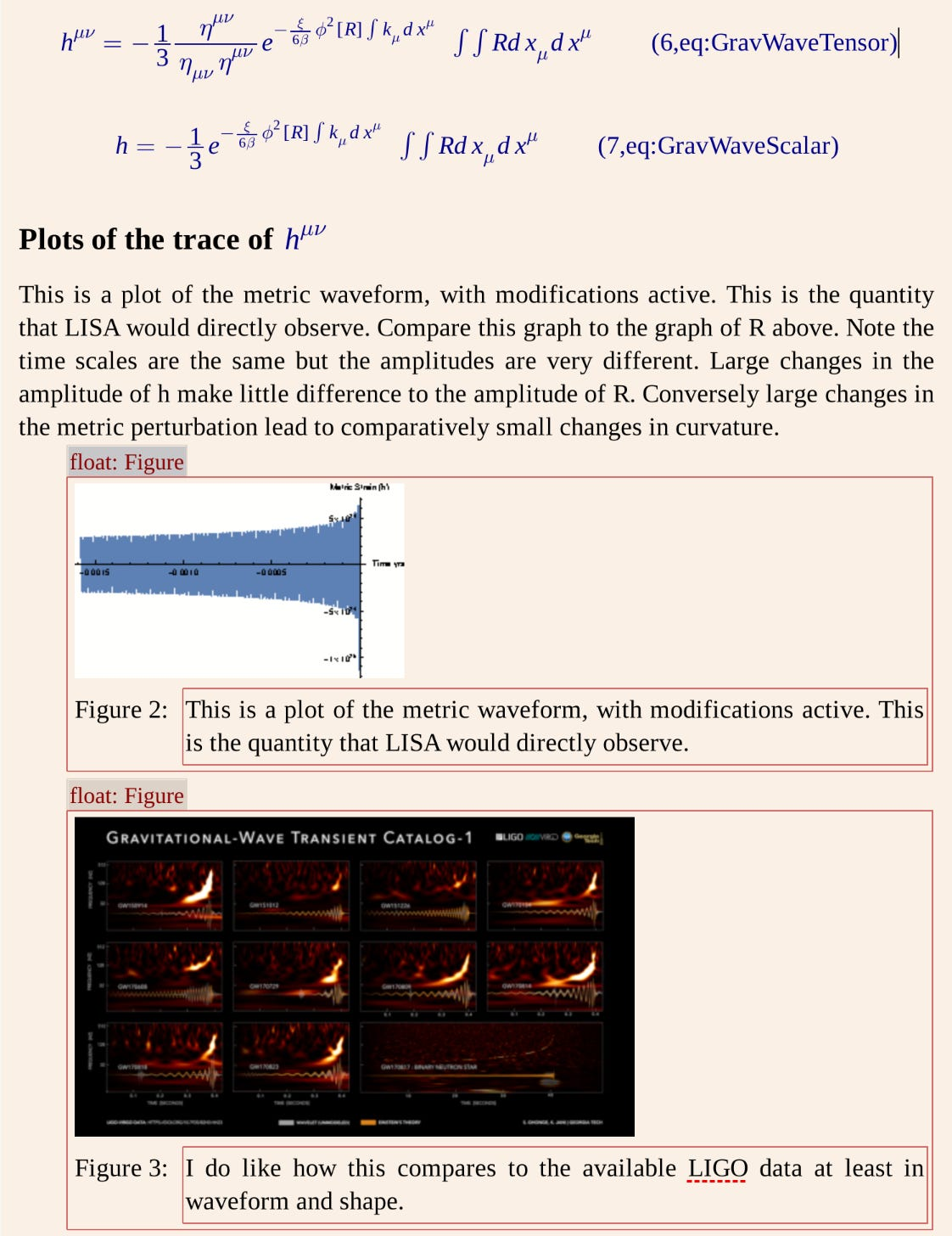The solution to the issue of dark matter and dark energy may be found by way of modifying Einstein's equations of General Relativity.  Working with Shane Larson of Northwestern University we may have found an analytical and exact solution to one such set of equations.  With this we may be able to test such proposals once and for all using the future Laser Interferometer Space Antenna, LISA. Exact solutions to the differential equations that describe fundamental physics are rare. So rare they are sometimes called by name.  As one of many collaborators in the Laser Interferometer Space Antenna, Fundamental Physics working group, solving such problems is just what I do. The work reported here is not the work of the working group, but I am guided by the group’s works and whitepapers. The result I report has not been peer reviewed. If it checks out, then we will be able to analyze questions of modified gravity without need for numerical approximation. Certain nonlinear terms that are beyond quadratic in the fields and which involve recursion relationships will still need to be analyzed with numerical methods.

In short we started with this which is known as the action of the theory.

Applied a lot of a type of math known as variation calculus to derive the field equations.  Then solve them up to an integral to find this.  Note the solution for the Ricci scalar contains a recursion.

The great Karl Schwarzschild said that we should avail ourselves of exact solutions of simple form, this solution is not simple. Solving the equation up to an integral is. The complicated part, which becomes pages and pages of Mathematica output isn’t.  Mathematica was used to check our math and also run numerical computations which when plotted found this.The general shape of which matches that of gravitational wave signatures seen from LIGO. LISA will look at a different range of frequencies than LIGO however so the time scale is different.

I include the results in the form of screen shots of a draft done in a program called LyX, an editor used to create LaTeX documents. This is a draft, not yet a pre print, of something that may or may not check out. Consider it a curious look at the life of a theoretical gravitational wave physicist.

### The Problem and Solution.

One idea of what dark matter could be is a very light scalar particle. These particles would be attracted to black holes. If these particles exist, then we should be able to tease out some proof of their existence via gravitational wave observations.

Several steps are not shown. The indicated derivatives had to be taken then the resulting equations simplified then solved up to doing what can be some complicated integrals. For example, the k that appears in the below is a killing vector appropriate for the problem.Which leads to the field equations that are to be solved. Through a long process which is not appropriate for a blogpost we arrive at the solutions.This looks deceptively simple. Note the Ricci scalar contains a recursive term in which it depends on itself in a very non linear way. That of course has to be handled with a computer. That said, this term can be discarded, and the results still match the observations LIGO has so far.

After several simplifying steps the metric perturbation, the change to the flat undisturbed space-time, which would be observed by gravitational wave observatories can be found.The plot of the solution VS the waveform. The characteristically “trumpet shaped” waveform is suggestive of correctness.

Theory and data match up to a tiny difference that would be the predicted modification due to the presence of a scalar field and the R squared term.